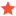Targeting from Camera Intel d435 Realsense95kvdkoJunior MemberPosts: 5 Threads: 3 Joined: Dec 2019 Reputation: 0 12-08-2019, 03:26 PM (This post was last modified: 12-09-2019, 08:10 PM by Albert. Edit Reason: Added Python code in a Code box ) Hi everyone, I'm doing a project where i want to click on a single point of an image and get the coordinates (x, y, z) of that point in real life. Then recreate on robodk the scenario of the camera and move a robotic arm (ur5e of universal robots) to that point. The thing is that if i print the coordinates (x, y, z) that i get from the camera, i think they make sense, but when i use them to create a target for robodk and move the arm there, they don't match with the point where should it go. This is the code that i'm using. Code:```import cv2                                # state of the art computer vision algorithms library import numpy as np                        # fundamental package for scientific computing import imageio                       import matplotlib.pyplot as plt           # 2D plotting library producing publication quality figures import pyrealsense2 as rs import win32gui import win32api import time import pyautogui import tkinter import tkinter.messagebox from robolink import *    # API to communicate with RoboDK from robodk import *      # basic matrix operations # Any interaction with RoboDK must be done through RL = Robolink() top = tkinter.Tk() points = rs.points() pipe = rs.pipeline() config = rs.config() profile = pipe.start(config) colorizer = rs.colorizer() align = rs.align(rs.stream.color) state_left = win32api.GetKeyState(0x01)  # Left button up = 0 or 1. Button down = -127 or -128 device = profile.get_device() # type: rs.device depth_sensor = device.first_depth_sensor() # type: rs.depth_sensor if depth_sensor.supports(rs.option.depth_units):  depth_sensor.set_option(rs.option.depth_units,0.0001) robot = RL.Item('UR5e') frame = RL.Item('Frame 2') Target = RL.AddTarget('Target 2', frame) try:    while True:       frames = pipe.wait_for_frames()       frames = align.process(frames)       depth_frame = frames.get_depth_frame()       color_frame = frames.get_color_frame()       if not depth_frame: continue       #print(width,height)       depth = np.asanyarray(colorizer.colorize(depth_frame).get_data())       color = np.asanyarray(color_frame.get_data())       images = np.hstack((color, depth))          #cv2.namedWindow('RGB_View', cv2.WINDOW_NORMAL)       #cv2.setWindowProperty('RGB_View', cv2.WND_PROP_FULLSCREEN, cv2.WINDOW_FULLSCREEN)       cv2.imshow('RGB_View', depth)       depth_intrin = depth_frame.profile.as_video_stream_profile().intrinsics       #flags, hcursor, (x,y) = win32gui.GetCursorInfo()       x, y = win32api.GetCursorPos()       #print(x, y)       pixel_coord = [x, y]       keypressed = win32api.GetKeyState(0x01)       key = cv2.waitKey(1)       # Press esc or 'q' to close the image window       if key & 0xFF == ord('q') or key == 27:           cv2.destroyAllWindows()           break       #Calculate distance       if keypressed!=state_left:        state_left = keypressed        print(keypressed)        if keypressed < 0:         if (0